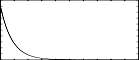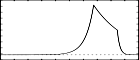## GEN05

GEN05 — Constructs functions from segments of exponential curves.

## Description

Constructs functions from segments of exponential curves.

## Syntax

`f # time size 5 a n1 b n2 c ...`

## Initialization

size -- number of points in the table. Must be a power of 2 or power-of-2 plus 1 (see f statement).

a, b, c, etc. -- ordinate values, in odd-numbered pfields p5, p7, p9, . . . These must be nonzero and must be alike in sign.

n1, n2, etc. -- length of segment (no. of storage locations), in even-numbered pfields. Cannot be negative, but a zero is meaningful for specifying discontinuous waveforms. The sum n1 + n2 + .... will normally equal size for fully specified functions. If the sum is smaller, the function locations not included will be set to zero; if the sum is greater, only the first size locations will be stored. Note that the values are rounded to integers before use.

NoteIf p4 is positive, functions are post-normalized (rescaled to a maximum absolute value of 1 after generation). A negative p4 will cause rescaling to be skipped. Discrete-point linear interpolation implies an increase or decrease along a segment by equal differences between adjacent locations; exponential interpolation implies that the progression is by equal ratio. In both forms the interpolation from a to b is such as to assume that the value b will be attained in the n + 1th location. For discontinuous functions, and for the segment encompassing the end location, this value will not actually be reached, although it may eventually appear as a result of final scaling.

## Examples

Here is a simple example of the GEN05 routine. It uses the files gen05.csd.

Example 1271. An example of the GEN05 routine.

```<CsoundSynthesizer>
<CsOptions>
; Select audio/midi flags here according to platform
-odac      ;;;realtime audio out
; For Non-realtime ouput leave only the line below:
; -o gen05.wav -W ;;; for file output any platform
</CsOptions>
<CsInstruments>

sr = 44100
ksmps = 32
nchnls = 2
0dbfs  = 1

instr 1

ifn = p4					;choose different tables for GEN05
kcps init 1/p3					;index over the length of  entire note
kndx phasor kcps
ixmode = 1					;normalize index data
kamp tablei kndx, ifn, ixmode
asig poscil kamp, 440, 1
outs asig, asig

endin
</CsInstruments>
<CsScore>
f 1 0 8192 10 1	;sine wave
f 2 0 129 5    1   100 0.0001 29 		;short attack
f 3 0 129 5 0.00001 87    1   22 .5 20 0.0001 	;long attack

i 1 0 2 2
i 1 3 2 3

e
</CsScore>
</CsoundSynthesizer>
```

These are the diagrams of the waveforms of the GEN05 routines, as used in the example:f 2 0 129 5 1 100 0.0001 29 - waveform that goes over 100 points from 1 to 0.0001, stay there for 29 pointsf 3 0 129 5 0.00001 87 1 22 .5 20 0.0001 - waveform that goes from 0.00001 to 1 in 87 points, then from 1 to .5 in 22 points and then from .5 to 0.0001 in 20 points